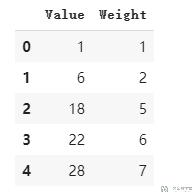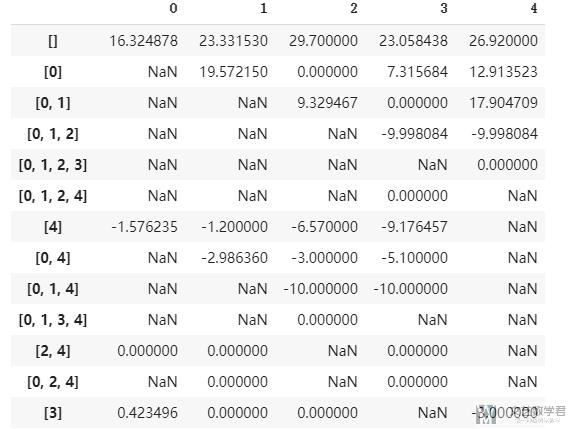# 强化学习解决背包问题2020年8月6日07:19:571 1 5870字阅读19分34秒

## 用强化学习解决背包问题

### 使用强化学习的想法

• state: 包里目前有的物体情况, 例如有物品1和物品2. 最后会保存为'[1,2]', 就表示包里有1号和2号物品.
• action: 有N种物品, 就有N中action. 把物品I放入包中, 就是执行动作I.
• reward: 每放进一个物体, 如果没有超重, reward是物品的value. 如果超重了, reward就是-10, 并且回合结束.

• 我们的想法就是从Q-table来入手. 当有新的状态添加到Q-table中的时候(比如现在状态是[1,3]), 我们就将action 1和action 3的位置设置为NaN, 这样在选max的时候, 就不会选到这些action. 这个在下面函数check_state中有进行实现.
• 同时我们还需要修改ϵ-greedy的函数. 因为里面有从所有动作里面随机挑一个, 所以我们要有一个列表, 这里存的是所有在当前state下, 可以挑选的动作. 这个具体的实现在函数mu_policy中进行实现.

## 具体代码实现

1. import pandas as pd
2. import numpy as np
3. import matplotlib.pyplot as plt
4. from time import time
5. import plotting
6. import itertools

### 初始化背包

1. item = pd.DataFrame(data=[[1, 1], [6, 2], [18, 5], [22, 6], [28, 7]], columns=['Value', 'Weight'])
2. actions = list(range(len(item))) # 动作, 每一个物体是一个动作
3. limit_W = 11 # 背包重量的限制
4. gamma = 0.9### 定义环境

1. def envReward(action, knapsack):
2.     """返回下一步的state, reward和done
3.     """
4.     limit_W = 11
5.     knapsack_ = knapsack + [action] # 得到新的背包里的东西, 现在是[2,3], 向里面增加物品, 得到新的状态[1,2,3]
6.     knapsack_.sort()
7.     knapsack_W = np.sum([item['Weight'][i] for i in knapsack_]) # 计算当前包内物品的总和
8.     if knapsack_W > limit_W:
9.         r = -10
10.         done = True
11.     else:
12.         r = item['Value'][action]
13.         done = False
14.     return r, knapsack_, done

### 策略的定义

• μ, 定义ϵ-greedy的策略;
• π, 定义greedy的策略;

1. def mu_policy(Q, epsilon, nA, observation, actions):
2.     """
3.     这是一个epsilon-greedy的策略, 返回每一个动作执行的概率, nA为动作的个数
4.     其中:
5.     - Q是q table, 为dataframe的格式;
6.     - nA是所有动作的个数
7.     """
8.     actionsList = list(set(actions).difference(set(observation))) # 可以挑选的动作
9.     # 看到这个state之后, 采取不同action获得的累计奖励
10.     action_values = Q.loc[str(observation), :]
11.     # 使用获得奖励最大的那个动作
12.     greedy_action = action_values.idxmax()
13.     # 是的每个动作都有出现的可能性
14.     probabilities = np.zeros(nA)
15.     for i in actionsList:
16.         probabilities[i] = 1/len(actionsList) * epsilon
17.     probabilities[greedy_action] = probabilities[greedy_action] + (1 - epsilon)
18.     return probabilities

1. def pi_policy(Q, observation):
2.     """
3.     这是greedy policy, 每次选择最优的动作.
4.     其中:
5.     - Q是q table, 为dataframe的格式;
6.     """
7.     action_values = Q.loc[str(observation), :]
8.     best_action = action_values.idxmax() # 选择最优的动作
9.     return np.eye(len(action_values))[best_action] # 返回的是两个动作出现的概率

### Q-table的检查

1. def check_state(Q, knapsack, actions):
2.     """检查状态knapsack是否在q-table中, 没有则进行添加
3.     """
4.     if str(knapsack) not in Q.index# knapsack表示状态, 例如现在包里有[1,2]
5.         # append new state to q table
6.         q_table_new = pd.Series([np.NAN]*len(actions), index=Q.columns, name=str(knapsack))
7.         # 下面是将能使用的状态设置为0, 不能使用的设置为NaN (这个很重要)
8.         for i in list(set(actions).difference(set(knapsack))):
9.             q_table_new[i] = 0
10.         return Q.append(q_table_new)
11.     else:
12.         return Q

### 开始更新Q-Table

1. def qLearning(actions, num_episodes, discount_factor=1.0, alpha=0.7, epsilon=0.2):
2.     # 环境中所有动作的数量
3.     nA = len(actions)
4.     # 初始化Q表
5.     Q = pd.DataFrame(columns=actions)
6.     # 记录reward和总长度的变化
7.     stats = plotting.EpisodeStats(
8.         episode_lengths=np.zeros(num_episodes+1),
9.         episode_rewards=np.zeros(num_episodes+1))
10.     for i_episode in range(1, num_episodes + 1):
11.         # 开始一轮游戏
12.         knapsack = [] # 开始的时候背包是空的
13.         Q = check_state(Q, knapsack, actions)
14.         action = np.random.choice(nA, p=mu_policy(Q, epsilon, nA, knapsack, actions)) # 从实际执行的policy, 选择action
15.         for t in itertools.count():
16.             reward, next_knapsack, done = envReward(action, knapsack) # 执行action, 返回reward和下一步的状态
17.             Q = check_state(Q, next_knapsack, actions)
18.             next_action = np.random.choice(nA, p=mu_policy(Q, epsilon, nA, next_knapsack, actions)) # 选择下一步的动作
19.             # 更新Q
20.             Q.loc[str(knapsack), action] = Q.loc[str(knapsack), action] + alpha*(reward + discount_factor*Q.loc[str(next_knapsack), :].max() - Q.loc[str(knapsack), action])
21.             # 计算统计数据(带有探索的策略)
22.             stats.episode_rewards[i_episode] += reward # 计算累计奖励
23.             stats.episode_lengths[i_episode] = t # 查看每一轮的时间
24.             if done:
25.                 break
26.             if t > 10:
27.                 break
28.             knapsack = next_knapsack
29.             action = next_action
30.         if i_episode % 50 == 0:
31.             # 打印
32.             print("\rEpisode {}/{}. | ".format(i_episode, num_episodes), end="")
33.     return Q, stats

1. Q, stats = qLearning(actions, num_episodes=1000, discount_factor=0.9, alpha=0.3, epsilon=0.1)### 结果测试

1. # 查看最终结果
2. actionsList = []
3. knapsack = [] # 开始的时候背包是空的
4. nA = len(actions)
5. action = np.random.choice(nA, p=pi_policy(Q, knapsack)) # 从实际执行的policy, 选择action
6. for t in itertools.count():
7.     actionsList.append(action)
8.     reward, next_knapsack, done = envReward(action, knapsack) # 执行action, 返回reward和下一步的状态
9.     next_action = np.random.choice(nA, p=pi_policy(Q, next_knapsack)) # 选择下一步的动作
10.     if done:
11.         actionsList.pop()
12.         break
13.     else:
14.         action = next_action
15.         knapsack = next_knapsack

• 微信公众号
• 关注微信公众号
•• QQ群
• 我们的QQ群号
•• 本文由 发表于 2020年8月6日07:19:57
• 转载请务必保留本文链接：https://mathpretty.com/12560.html

•guava

您好，想請問若想解決的是多目標背包問題的話，例如有N個物品要放入P個背包，這個方法是否可行或是能用何種方法解決，謝謝。